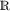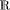# 8.1. Generalities

A statistical modelis said to be non-parametric if Θ is “vast”. When Θ is a vector space or a convex set, “vast” generally means “of infinite dimension”, otherwise the distinction between parametric and non-parametric models is not so clear.

EXAMPLE 8.1.–

1) A Gaussian or exponential model is parametric.

2) Let P0 be the set of probabilities ondominated by the Lebesgue measure λ. The modelis non-parametric (we may set θ = dP/dλ, and Θ is a convex set of infinite dimension in L1(λ)).

3) Let P1 be the set of probabilities onwhich have a unique median. The modelis non-parametric.

Non-parametric methods are interesting for three principal reasons:

1) They avoid errors due to the choice of a specific but often erroneous parametric model.

2) They guide the user in the choice of a parametric model.

3) In certain cases, they provide initial estimators for the parameters of a parametric model from which we may construct more ...

Get Mathematical Statistics and Stochastic Processes now with the O’Reilly learning platform.

O’Reilly members experience books, live events, courses curated by job role, and more from O’Reilly and nearly 200 top publishers.